Anzeige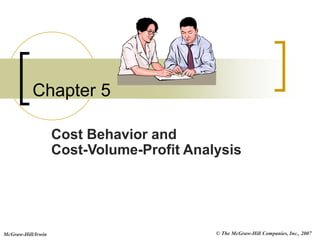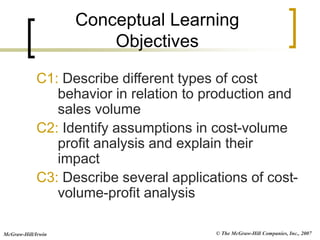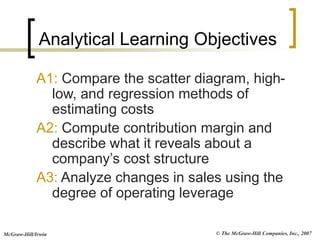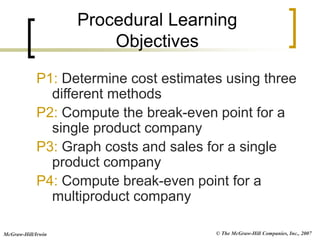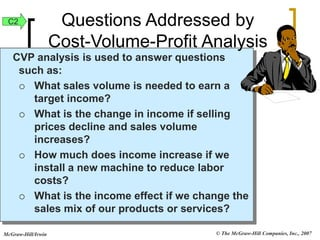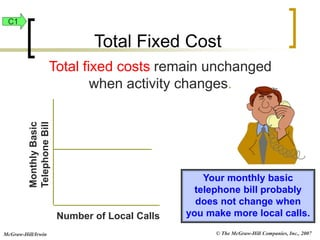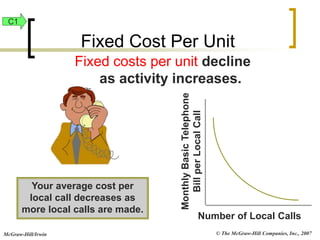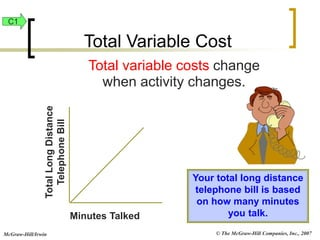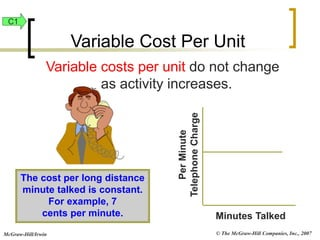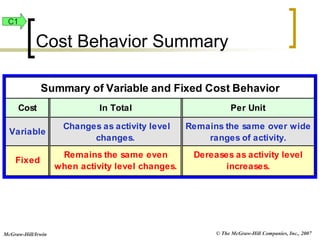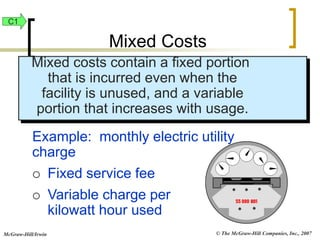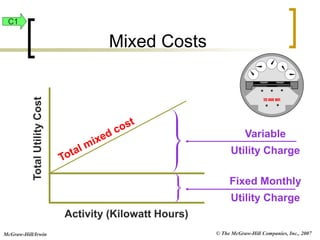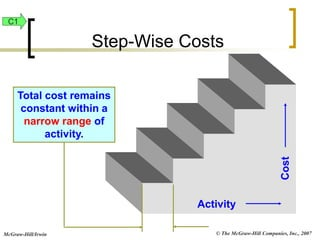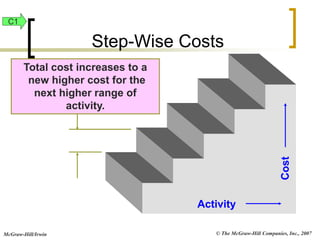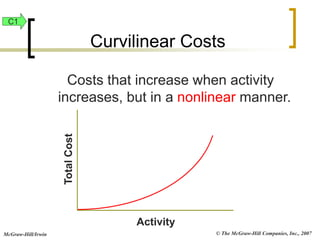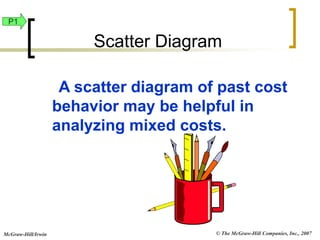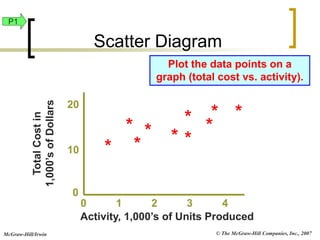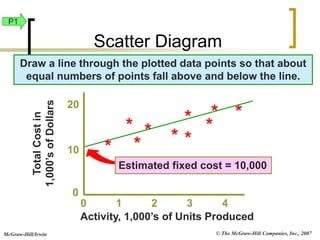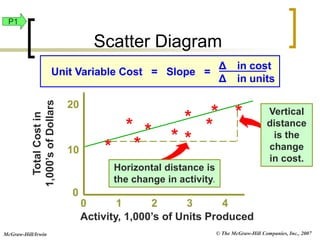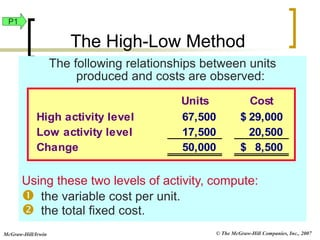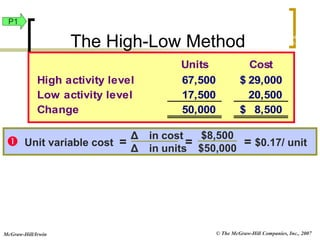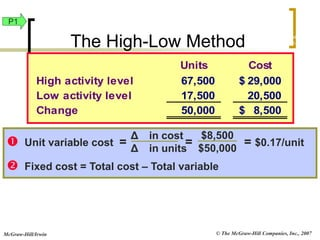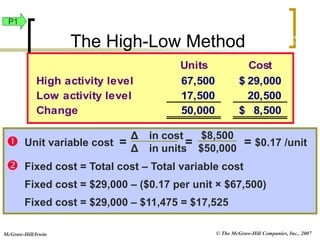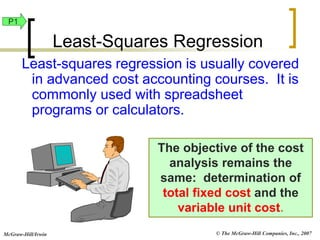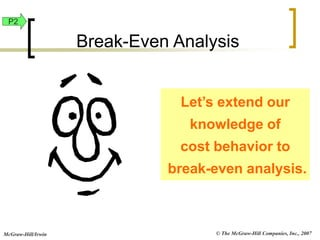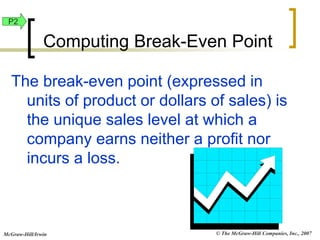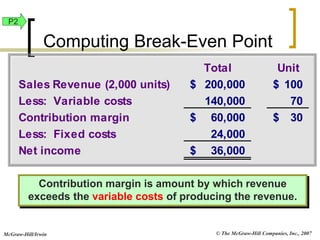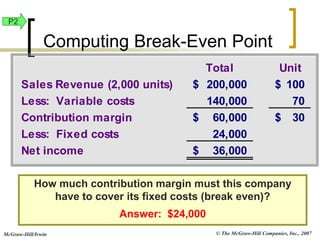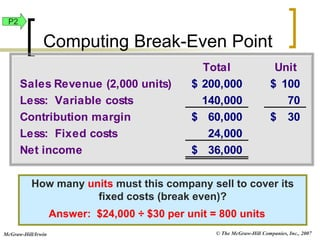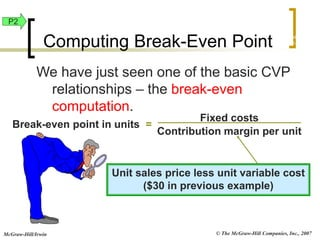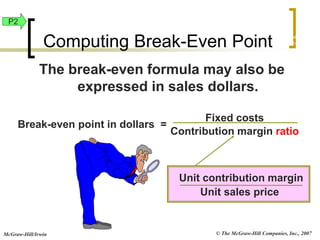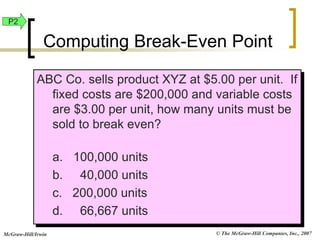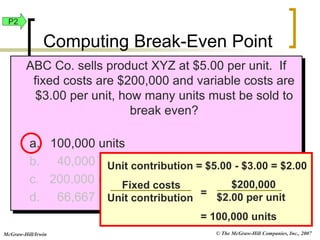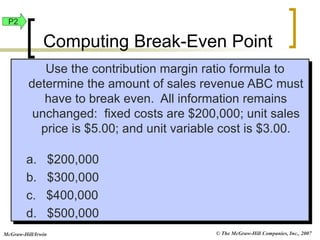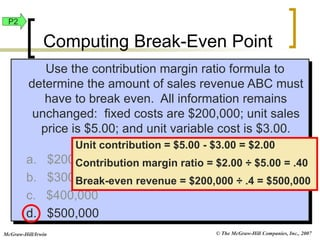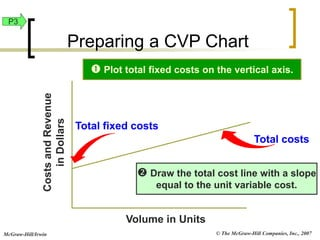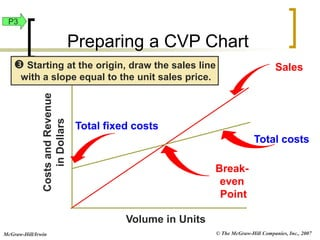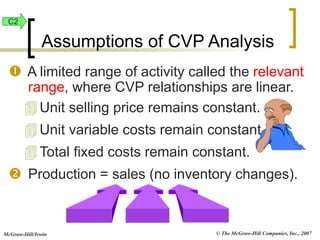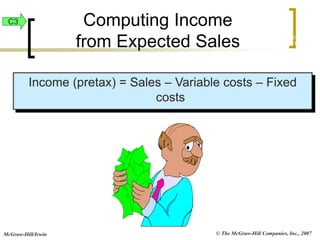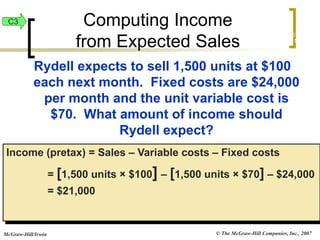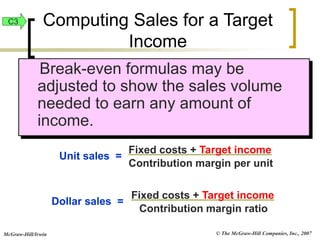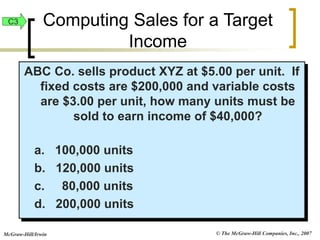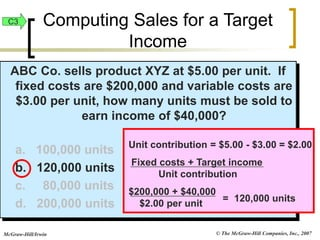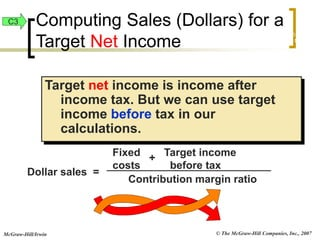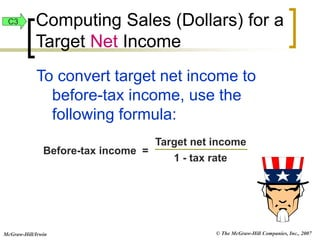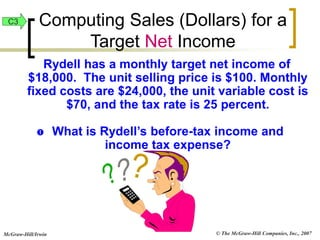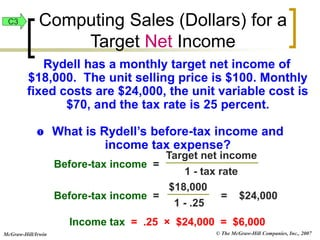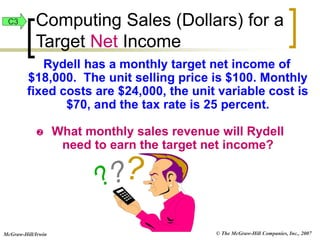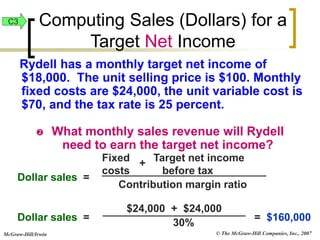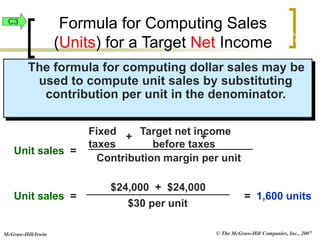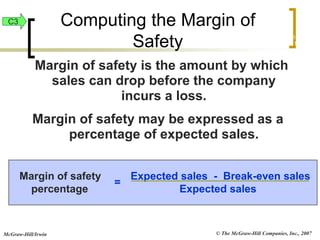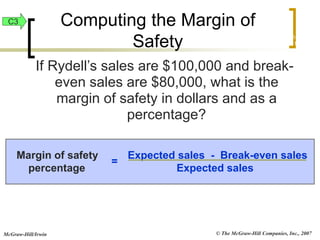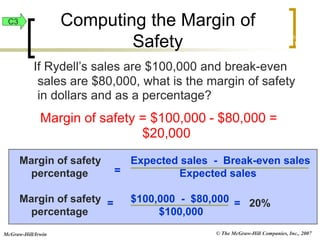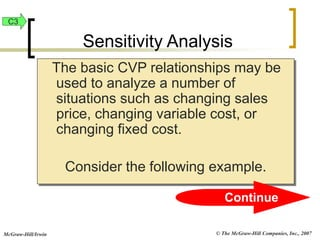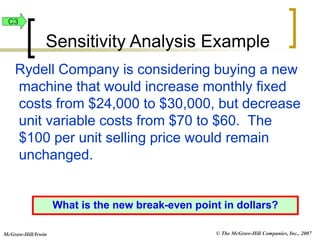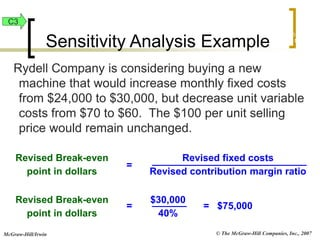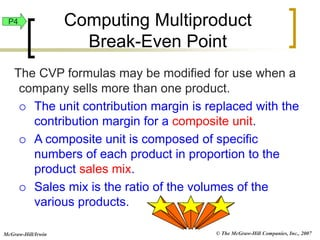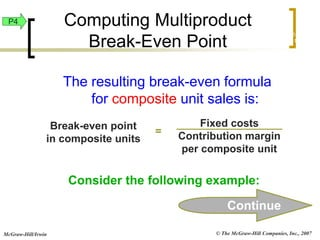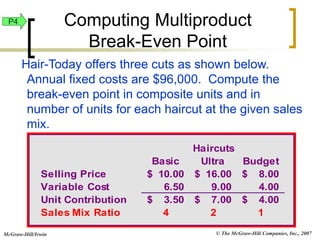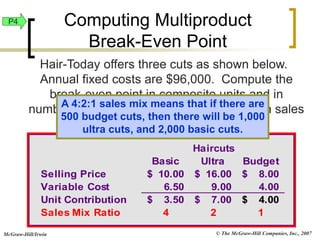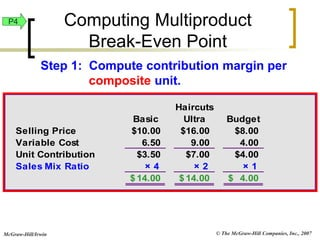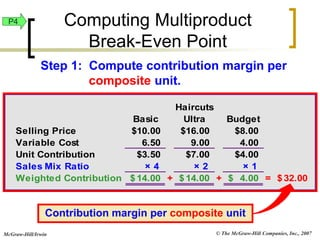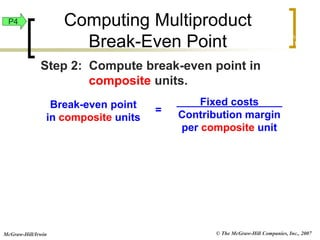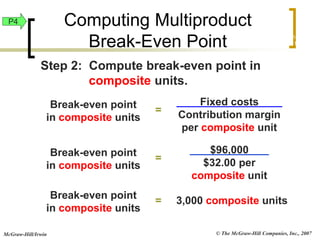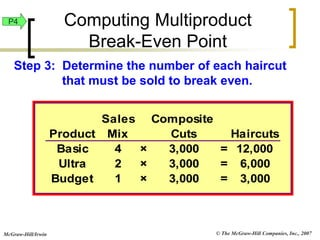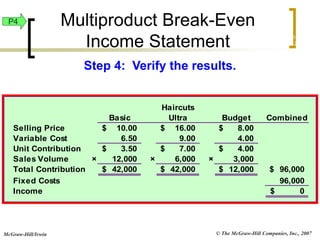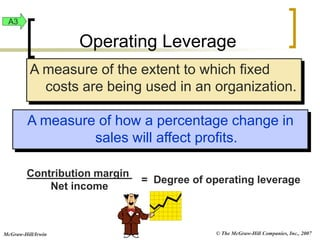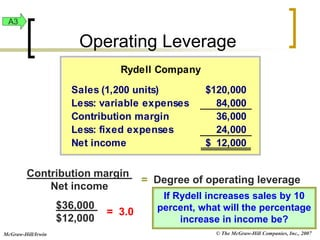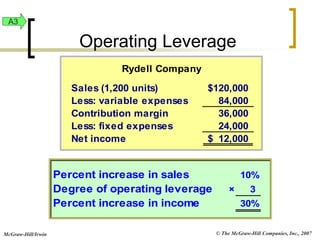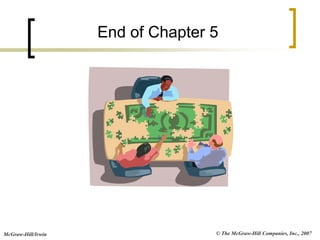1 von 71
Anzeige

### yrtyt

1. © The McGraw-Hill Companies, Inc., 2007 McGraw-Hill/Irwin Chapter 5 Cost Behavior and Cost-Volume-Profit Analysis
2. © The McGraw-Hill Companies, Inc., 2007 McGraw-Hill/Irwin Conceptual Learning Objectives C1: Describe different types of cost behavior in relation to production and sales volume C2: Identify assumptions in cost-volume profit analysis and explain their impact C3: Describe several applications of cost- volume-profit analysis
3. © The McGraw-Hill Companies, Inc., 2007 McGraw-Hill/Irwin A1: Compare the scatter diagram, high- low, and regression methods of estimating costs A2: Compute contribution margin and describe what it reveals about a company’s cost structure A3: Analyze changes in sales using the degree of operating leverage Analytical Learning Objectives
4. © The McGraw-Hill Companies, Inc., 2007 McGraw-Hill/Irwin P1: Determine cost estimates using three different methods P2: Compute the break-even point for a single product company P3: Graph costs and sales for a single product company P4: Compute break-even point for a multiproduct company Procedural Learning Objectives
5. © The McGraw-Hill Companies, Inc., 2007 McGraw-Hill/Irwin CVP analysis is used to answer questions such as:  What sales volume is needed to earn a target income?  What is the change in income if selling prices decline and sales volume increases?  How much does income increase if we install a new machine to reduce labor costs?  What is the income effect if we change the sales mix of our products or services? Questions Addressed by Cost-Volume-Profit Analysis C2
6. © The McGraw-Hill Companies, Inc., 2007 McGraw-Hill/Irwin Number of Local Calls Monthly Basic Telephone Bill Total fixed costs remain unchanged when activity changes. Your monthly basic telephone bill probably does not change when you make more local calls. Total Fixed Cost C1
7. © The McGraw-Hill Companies, Inc., 2007 McGraw-Hill/Irwin Number of Local Calls Monthly Basic Telephone Bill per Local Call Fixed costs per unit decline as activity increases. Your average cost per local call decreases as more local calls are made. Fixed Cost Per Unit C1
8. © The McGraw-Hill Companies, Inc., 2007 McGraw-Hill/Irwin Minutes Talked Total Long Distance Telephone Bill Total variable costs change when activity changes. Your total long distance telephone bill is based on how many minutes you talk. Total Variable Cost C1
9. © The McGraw-Hill Companies, Inc., 2007 McGraw-Hill/Irwin Minutes Talked Per Minute Telephone Charge Variable costs per unit do not change as activity increases. The cost per long distance minute talked is constant. For example, 7 cents per minute. Variable Cost Per Unit C1
10. © The McGraw-Hill Companies, Inc., 2007 McGraw-Hill/Irwin Summary of Variable and Fixed Cost Behavior Cost In Total Per Unit Variable Changes as activity level changes. Remains the same over wide ranges of activity. Fixed Remains the same even when activity level changes. Dereases as activity level increases. Cost Behavior Summary C1
11. © The McGraw-Hill Companies, Inc., 2007 McGraw-Hill/Irwin Mixed costs contain a fixed portion that is incurred even when the facility is unused, and a variable portion that increases with usage. Example: monthly electric utility charge  Fixed service fee  Variable charge per kilowatt hour used Mixed Costs C1
12. © The McGraw-Hill Companies, Inc., 2007 McGraw-Hill/Irwin Variable Utility Charge Activity (Kilowatt Hours) Total Utility Cost Fixed Monthly Utility Charge Mixed Costs C1
13. © The McGraw-Hill Companies, Inc., 2007 McGraw-Hill/Irwin Activity Cost Total cost remains constant within a narrow range of activity. Step-Wise Costs C1
14. © The McGraw-Hill Companies, Inc., 2007 McGraw-Hill/Irwin Activity Cost Total cost increases to a new higher cost for the next higher range of activity. Step-Wise Costs C1
15. © The McGraw-Hill Companies, Inc., 2007 McGraw-Hill/Irwin Costs that increase when activity increases, but in a nonlinear manner. Activity Total Cost Curvilinear Costs C1
16. © The McGraw-Hill Companies, Inc., 2007 McGraw-Hill/Irwin The objective is to classify all costs as either fixed or variable. Identifying and Measuring Cost Behavior P1
17. © The McGraw-Hill Companies, Inc., 2007 McGraw-Hill/Irwin A scatter diagram of past cost behavior may be helpful in analyzing mixed costs. Scatter Diagram P1
18. © The McGraw-Hill Companies, Inc., 2007 McGraw-Hill/Irwin Plot the data points on a graph (total cost vs. activity). 0 1 2 3 4 * Total Cost in 1,000’s of Dollars 10 20 0 * * * * * * * * * Activity, 1,000’s of Units Produced P1 Scatter Diagram
19. © The McGraw-Hill Companies, Inc., 2007 McGraw-Hill/Irwin Draw a line through the plotted data points so that about equal numbers of points fall above and below the line. Estimated fixed cost = 10,000 0 1 2 3 4 * Total Cost in 1,000’s of Dollars 10 20 0 * * * * * * * * * Activity, 1,000’s of Units Produced P1 Scatter Diagram
20. © The McGraw-Hill Companies, Inc., 2007 McGraw-Hill/Irwin Vertical distance is the change in cost. Horizontal distance is the change in activity. Unit Variable Cost = Slope = Δ in cost Δ in units 0 1 2 3 4 * Total Cost in 1,000’s of Dollars 10 20 0 * * * * * * * * * Activity, 1,000’s of Units Produced P1 Scatter Diagram
21. © The McGraw-Hill Companies, Inc., 2007 McGraw-Hill/Irwin The following relationships between units produced and costs are observed: Using these two levels of activity, compute:  the variable cost per unit.  the total fixed cost. Units Cost High activity level 67,500 29,000 \$ Low activity level 17,500 20,500 Change 50,000 8,500 \$ The High-Low Method P1
22. © The McGraw-Hill Companies, Inc., 2007 McGraw-Hill/Irwin  Unit variable cost = = = \$0.17/ unit Δ in cost Δ in units \$8,500 \$50,000 Units Cost High activity level 67,500 29,000 \$ Low activity level 17,500 20,500 Change 50,000 8,500 \$ Exh. 22-6 P1 The High-Low Method
23. © The McGraw-Hill Companies, Inc., 2007 McGraw-Hill/Irwin Units Cost High activity level 67,500 29,000 \$ Low activity level 17,500 20,500 Change 50,000 8,500 \$  Unit variable cost = = = \$0.17/unit  Fixed cost = Total cost – Total variable Δ in cost Δ in units \$8,500 \$50,000 Exh. 22-6 P1 The High-Low Method
24. © The McGraw-Hill Companies, Inc., 2007 McGraw-Hill/Irwin Units Cost High activity level 67,500 29,000 \$ Low activity level 17,500 20,500 Change 50,000 8,500 \$  Unit variable cost = = = \$0.17 /unit  Fixed cost = Total cost – Total variable cost Fixed cost = \$29,000 – (\$0.17 per unit × \$67,500) Fixed cost = \$29,000 – \$11,475 = \$17,525 Δ in cost Δ in units \$8,500 \$50,000 Exh. 22-6 P1 The High-Low Method
25. © The McGraw-Hill Companies, Inc., 2007 McGraw-Hill/Irwin The objective of the cost analysis remains the same: determination of total fixed cost and the variable unit cost. Least-squares regression is usually covered in advanced cost accounting courses. It is commonly used with spreadsheet programs or calculators. Least-Squares Regression P1
26. © The McGraw-Hill Companies, Inc., 2007 McGraw-Hill/Irwin Let’s extend our knowledge of cost behavior to break-even analysis. Break-Even Analysis P2
27. © The McGraw-Hill Companies, Inc., 2007 McGraw-Hill/Irwin The break-even point (expressed in units of product or dollars of sales) is the unique sales level at which a company earns neither a profit nor incurs a loss. Computing Break-Even Point P2
28. © The McGraw-Hill Companies, Inc., 2007 McGraw-Hill/Irwin Contribution margin is amount by which revenue exceeds the variable costs of producing the revenue. Total Unit Sales Revenue (2,000 units) 200,000 \$ 100 \$ Less: Variable costs 140,000 70 Contribution margin 60,000 \$ 30 \$ Less: Fixed costs 24,000 Net income 36,000 \$ Computing Break-Even Point P2
29. © The McGraw-Hill Companies, Inc., 2007 McGraw-Hill/Irwin Total Unit Sales Revenue (2,000 units) 200,000 \$ 100 \$ Less: Variable costs 140,000 70 Contribution margin 60,000 \$ 30 \$ Less: Fixed costs 24,000 Net income 36,000 \$ How much contribution margin must this company have to cover its fixed costs (break even)? Answer: \$24,000 P2 Computing Break-Even Point
30. © The McGraw-Hill Companies, Inc., 2007 McGraw-Hill/Irwin How many units must this company sell to cover its fixed costs (break even)? Total Unit Sales Revenue (2,000 units) 200,000 \$ 100 \$ Less: Variable costs 140,000 70 Contribution margin 60,000 \$ 30 \$ Less: Fixed costs 24,000 Net income 36,000 \$ Answer: \$24,000 ÷ \$30 per unit = 800 units P2 Computing Break-Even Point
31. © The McGraw-Hill Companies, Inc., 2007 McGraw-Hill/Irwin We have just seen one of the basic CVP relationships – the break-even computation. Break-even point in units = Fixed costs Contribution margin per unit Computing Break-Even Point Unit sales price less unit variable cost (\$30 in previous example) Exh. 22-8 P2
32. © The McGraw-Hill Companies, Inc., 2007 McGraw-Hill/Irwin The break-even formula may also be expressed in sales dollars. Break-even point in dollars = Fixed costs Contribution margin ratio Unit contribution margin Unit sales price Exh. 22-9 P2 Computing Break-Even Point
33. © The McGraw-Hill Companies, Inc., 2007 McGraw-Hill/Irwin ABC Co. sells product XYZ at \$5.00 per unit. If fixed costs are \$200,000 and variable costs are \$3.00 per unit, how many units must be sold to break even? a. 100,000 units b. 40,000 units c. 200,000 units d. 66,667 units P2 Computing Break-Even Point
34. © The McGraw-Hill Companies, Inc., 2007 McGraw-Hill/Irwin ABC Co. sells product XYZ at \$5.00 per unit. If fixed costs are \$200,000 and variable costs are \$3.00 per unit, how many units must be sold to break even? a. 100,000 units b. 40,000 units c. 200,000 units d. 66,667 units Unit contribution = \$5.00 - \$3.00 = \$2.00 Fixed costs Unit contribution = \$200,000 \$2.00 per unit = 100,000 units P2 Computing Break-Even Point
35. © The McGraw-Hill Companies, Inc., 2007 McGraw-Hill/Irwin Use the contribution margin ratio formula to determine the amount of sales revenue ABC must have to break even. All information remains unchanged: fixed costs are \$200,000; unit sales price is \$5.00; and unit variable cost is \$3.00. a. \$200,000 b. \$300,000 c. \$400,000 d. \$500,000 P2 Computing Break-Even Point
36. © The McGraw-Hill Companies, Inc., 2007 McGraw-Hill/Irwin Use the contribution margin ratio formula to determine the amount of sales revenue ABC must have to break even. All information remains unchanged: fixed costs are \$200,000; unit sales price is \$5.00; and unit variable cost is \$3.00. a. \$200,000 b. \$300,000 c. \$400,000 d. \$500,000 Unit contribution = \$5.00 - \$3.00 = \$2.00 Contribution margin ratio = \$2.00 ÷ \$5.00 = .40 Break-even revenue = \$200,000 ÷ .4 = \$500,000 P2 Computing Break-Even Point
37. © The McGraw-Hill Companies, Inc., 2007 McGraw-Hill/Irwin Volume in Units Costs and Revenue in Dollars Total fixed costs Total costs  Draw the total cost line with a slope equal to the unit variable cost.  Plot total fixed costs on the vertical axis. Preparing a CVP Chart P3
38. © The McGraw-Hill Companies, Inc., 2007 McGraw-Hill/Irwin Sales Volume in Units Costs and Revenue in Dollars  Starting at the origin, draw the sales line with a slope equal to the unit sales price. Preparing a CVP Chart Break- even Point Total costs Total fixed costs P3
39. © The McGraw-Hill Companies, Inc., 2007 McGraw-Hill/Irwin  A limited range of activity called the relevant range, where CVP relationships are linear. Unit selling price remains constant. Unit variable costs remain constant. Total fixed costs remain constant.  Production = sales (no inventory changes). Assumptions of CVP Analysis C2
40. © The McGraw-Hill Companies, Inc., 2007 McGraw-Hill/Irwin Income (pretax) = Sales – Variable costs – Fixed costs Computing Income from Expected Sales Exh. 22-12 C3
41. © The McGraw-Hill Companies, Inc., 2007 McGraw-Hill/Irwin Rydell expects to sell 1,500 units at \$100 each next month. Fixed costs are \$24,000 per month and the unit variable cost is \$70. What amount of income should Rydell expect? Income (pretax) = Sales – Variable costs – Fixed costs = [1,500 units × \$100] – [1,500 units × \$70] – \$24,000 = \$21,000 Computing Income from Expected Sales Exh. 22-13 C3
42. © The McGraw-Hill Companies, Inc., 2007 McGraw-Hill/Irwin Break-even formulas may be adjusted to show the sales volume needed to earn any amount of income. Unit sales = Fixed costs + Target income Contribution margin per unit Dollar sales = Fixed costs + Target income Contribution margin ratio Computing Sales for a Target Income C3
43. © The McGraw-Hill Companies, Inc., 2007 McGraw-Hill/Irwin ABC Co. sells product XYZ at \$5.00 per unit. If fixed costs are \$200,000 and variable costs are \$3.00 per unit, how many units must be sold to earn income of \$40,000? a. 100,000 units b. 120,000 units c. 80,000 units d. 200,000 units C3 Computing Sales for a Target Income
44. © The McGraw-Hill Companies, Inc., 2007 McGraw-Hill/Irwin ABC Co. sells product XYZ at \$5.00 per unit. If fixed costs are \$200,000 and variable costs are \$3.00 per unit, how many units must be sold to earn income of \$40,000? a. 100,000 units b. 120,000 units c. 80,000 units d. 200,000 units = 120,000 units Unit contribution = \$5.00 - \$3.00 = \$2.00 Fixed costs + Target income Unit contribution \$200,000 + \$40,000 \$2.00 per unit C3 Computing Sales for a Target Income
45. © The McGraw-Hill Companies, Inc., 2007 McGraw-Hill/Irwin Target net income is income after income tax. But we can use target income before tax in our calculations. Dollar sales = Fixed Target income costs before tax Contribution margin ratio + Computing Sales (Dollars) for a Target Net Income Exh. 22-14 C3
46. © The McGraw-Hill Companies, Inc., 2007 McGraw-Hill/Irwin To convert target net income to before-tax income, use the following formula: Before-tax income = Target net income 1 - tax rate C3 Computing Sales (Dollars) for a Target Net Income
47. © The McGraw-Hill Companies, Inc., 2007 McGraw-Hill/Irwin Rydell has a monthly target net income of \$18,000. The unit selling price is \$100. Monthly fixed costs are \$24,000, the unit variable cost is \$70, and the tax rate is 25 percent.  What is Rydell’s before-tax income and income tax expense? C3 Computing Sales (Dollars) for a Target Net Income
48. © The McGraw-Hill Companies, Inc., 2007 McGraw-Hill/Irwin Before-tax income = Target net income 1 - tax rate Before-tax income = = \$24,000 \$18,000 1 - .25 Income tax = .25 × \$24,000 = \$6,000 Rydell has a monthly target net income of \$18,000. The unit selling price is \$100. Monthly fixed costs are \$24,000, the unit variable cost is \$70, and the tax rate is 25 percent.  What is Rydell’s before-tax income and income tax expense? C3 Computing Sales (Dollars) for a Target Net Income
49. © The McGraw-Hill Companies, Inc., 2007 McGraw-Hill/Irwin Rydell has a monthly target net income of \$18,000. The unit selling price is \$100. Monthly fixed costs are \$24,000, the unit variable cost is \$70, and the tax rate is 25 percent.  What monthly sales revenue will Rydell need to earn the target net income? C3 Computing Sales (Dollars) for a Target Net Income
50. © The McGraw-Hill Companies, Inc., 2007 McGraw-Hill/Irwin Dollar sales = Fixed Target net income costs before tax Contribution margin ratio + Dollar sales = = \$160,000 \$24,000 + \$24,000 30% Rydell has a monthly target net income of \$18,000. The unit selling price is \$100. Monthly fixed costs are \$24,000, the unit variable cost is \$70, and the tax rate is 25 percent.  What monthly sales revenue will Rydell need to earn the target net income? C3 Computing Sales (Dollars) for a Target Net Income
51. © The McGraw-Hill Companies, Inc., 2007 McGraw-Hill/Irwin The formula for computing dollar sales may be used to compute unit sales by substituting contribution per unit in the denominator. Contribution margin per unit Unit sales = Fixed Target net income taxes before taxes + + Unit sales = = 1,600 units \$24,000 + \$24,000 \$30 per unit Formula for Computing Sales (Units) for a Target Net Income Exh. 22-16 C3
52. © The McGraw-Hill Companies, Inc., 2007 McGraw-Hill/Irwin Margin of safety is the amount by which sales can drop before the company incurs a loss. Margin of safety may be expressed as a percentage of expected sales. Computing the Margin of Safety Exh. 22-17 Margin of safety Expected sales - Break-even sales percentage Expected sales = C3
53. © The McGraw-Hill Companies, Inc., 2007 McGraw-Hill/Irwin Margin of safety Expected sales - Break-even sales percentage Expected sales = If Rydell’s sales are \$100,000 and break- even sales are \$80,000, what is the margin of safety in dollars and as a percentage? Exh. 22-17 C3 Computing the Margin of Safety
54. © The McGraw-Hill Companies, Inc., 2007 McGraw-Hill/Irwin If Rydell’s sales are \$100,000 and break-even sales are \$80,000, what is the margin of safety in dollars and as a percentage? Margin of safety = \$100,000 - \$80,000 = \$20,000 Margin of safety Expected sales - Break-even sales percentage Expected sales = Margin of safety \$100,000 - \$80,000 percentage \$100,000 = = 20% Exh. 22-17 C3 Computing the Margin of Safety
55. © The McGraw-Hill Companies, Inc., 2007 McGraw-Hill/Irwin The basic CVP relationships may be used to analyze a number of situations such as changing sales price, changing variable cost, or changing fixed cost. Consider the following example. Continue Sensitivity Analysis C3
56. © The McGraw-Hill Companies, Inc., 2007 McGraw-Hill/Irwin Rydell Company is considering buying a new machine that would increase monthly fixed costs from \$24,000 to \$30,000, but decrease unit variable costs from \$70 to \$60. The \$100 per unit selling price would remain unchanged. What is the new break-even point in dollars? Sensitivity Analysis Example C3
57. © The McGraw-Hill Companies, Inc., 2007 McGraw-Hill/Irwin Rydell Company is considering buying a new machine that would increase monthly fixed costs from \$24,000 to \$30,000, but decrease unit variable costs from \$70 to \$60. The \$100 per unit selling price would remain unchanged. Revised Break-even point in dollars Revised fixed costs Revised contribution margin ratio Revised Break-even point in dollars \$30,000 40% = \$75,000 = = Exh. 22-18 C3 Sensitivity Analysis Example
58. © The McGraw-Hill Companies, Inc., 2007 McGraw-Hill/Irwin The CVP formulas may be modified for use when a company sells more than one product.  The unit contribution margin is replaced with the contribution margin for a composite unit.  A composite unit is composed of specific numbers of each product in proportion to the product sales mix.  Sales mix is the ratio of the volumes of the various products. Computing Multiproduct Break-Even Point P4
59. © The McGraw-Hill Companies, Inc., 2007 McGraw-Hill/Irwin The resulting break-even formula for composite unit sales is: Break-even point in composite units Fixed costs Contribution margin per composite unit = Consider the following example: Continue Exh. 22-19 P4 Computing Multiproduct Break-Even Point
60. © The McGraw-Hill Companies, Inc., 2007 McGraw-Hill/Irwin Hair-Today offers three cuts as shown below. Annual fixed costs are \$96,000. Compute the break-even point in composite units and in number of units for each haircut at the given sales mix. Haircuts Basic Ultra Budget Selling Price 10.00 \$ 16.00 \$ 8.00 \$ Variable Cost 6.50 9.00 4.00 Unit Contribution 3.50 \$ 7.00 \$ 4.00 \$ Sales Mix Ratio 4 2 1 P4 Computing Multiproduct Break-Even Point
61. © The McGraw-Hill Companies, Inc., 2007 McGraw-Hill/Irwin Hair-Today offers three cuts as shown below. Annual fixed costs are \$96,000. Compute the break-even point in composite units and in number of units for each haircut at the given sales mix. Haircuts Basic Ultra Budget Selling Price 10.00 \$ 16.00 \$ 8.00 \$ Variable Cost 6.50 9.00 4.00 Unit Contribution 3.50 \$ 7.00 \$ 4.00 \$ Sales Mix Ratio 4 2 1 A 4:2:1 sales mix means that if there are 500 budget cuts, then there will be 1,000 ultra cuts, and 2,000 basic cuts. P4 Computing Multiproduct Break-Even Point
62. © The McGraw-Hill Companies, Inc., 2007 McGraw-Hill/Irwin Haircuts Basic Ultra Budget Selling Price \$10.00 \$16.00 \$8.00 Variable Cost 6.50 9.00 4.00 Unit Contribution \$3.50 \$7.00 \$4.00 Sales Mix Ratio × 4 × 2 × 1 14.00 \$ 14.00 \$ 4.00 \$ Step 1: Compute contribution margin per composite unit. P4 Computing Multiproduct Break-Even Point
63. © The McGraw-Hill Companies, Inc., 2007 McGraw-Hill/Irwin Haircuts Basic Ultra Budget Selling Price \$10.00 \$16.00 \$8.00 Variable Cost 6.50 9.00 4.00 Unit Contribution \$3.50 \$7.00 \$4.00 Sales Mix Ratio × 4 × 2 × 1 Weighted Contribution 14.00 \$ + 14.00 \$ + 4.00 \$ = 32.00 \$ Contribution margin per composite unit Step 1: Compute contribution margin per composite unit. P4 Computing Multiproduct Break-Even Point
64. © The McGraw-Hill Companies, Inc., 2007 McGraw-Hill/Irwin Break-even point in composite units Fixed costs Contribution margin per composite unit = Step 2: Compute break-even point in composite units. Exh. 22-19 P4 Computing Multiproduct Break-Even Point
65. © The McGraw-Hill Companies, Inc., 2007 McGraw-Hill/Irwin Break-even point in composite units Fixed costs Contribution margin per composite unit = Step 2: Compute break-even point in composite units. Break-even point in composite units \$96,000 \$32.00 per composite unit = Break-even point in composite units = 3,000 composite units Exh. 22-19 P4 Computing Multiproduct Break-Even Point
66. © The McGraw-Hill Companies, Inc., 2007 McGraw-Hill/Irwin Sales Composite Product Mix Cuts Haircuts Basic 4 × 3,000 = 12,000 Ultra 2 × 3,000 = 6,000 Budget 1 × 3,000 = 3,000 Step 3: Determine the number of each haircut that must be sold to break even. P4 Computing Multiproduct Break-Even Point
67. © The McGraw-Hill Companies, Inc., 2007 McGraw-Hill/Irwin Haircuts Basic Ultra Budget Combined Selling Price 10.00 \$ 16.00 \$ 8.00 \$ Variable Cost 6.50 9.00 4.00 Unit Contribution 3.50 \$ 7.00 \$ 4.00 \$ Sales Volume × 12,000 × 6,000 × 3,000 Total Contribution 42,000 \$ 42,000 \$ 12,000 \$ 96,000 \$ Fixed Costs 96,000 Income \$ 0 Step 4: Verify the results. Multiproduct Break-Even Income Statement Exh. 22-20 P4
68. © The McGraw-Hill Companies, Inc., 2007 McGraw-Hill/Irwin A measure of the extent to which fixed costs are being used in an organization. A measure of how a percentage change in sales will affect profits. Contribution margin Net income = Degree of operating leverage Operating Leverage A3
69. © The McGraw-Hill Companies, Inc., 2007 McGraw-Hill/Irwin Rydell Company Sales (1,200 units) 120,000 \$ Less: variable expenses 84,000 Contribution margin 36,000 Less: fixed expenses 24,000 Net income 12,000 \$ \$36,000 \$12,000 = 3.0 Contribution margin Net income = Degree of operating leverage If Rydell increases sales by 10 percent, what will the percentage increase in income be? Operating Leverage A3
70. © The McGraw-Hill Companies, Inc., 2007 McGraw-Hill/Irwin Percent increase in sales 10% Degree of operating leverage × 3 Percent increase in income 30% Operating Leverage Rydell Company Sales (1,200 units) 120,000 \$ Less: variable expenses 84,000 Contribution margin 36,000 Less: fixed expenses 24,000 Net income 12,000 \$ A3
71. © The McGraw-Hill Companies, Inc., 2007 McGraw-Hill/Irwin End of Chapter 5

### Hinweis der Redaktion

1. This chapter shows how information on both costs and sales behavior is useful to managers in performing cost-volume-profit analysis. This analysis is an important part of successful management and sound business decisions.
2. Cost-volume-profit analysis will allow us to answer many questions and make important decisions involving the relationships between the volume of activity and costs and revenues. Before we can answer these questions using cost-volume-profit analysis, we must first study cost behavior.
3. We begin our study of cost behavior with fixed costs. Your basic land-line telephone has a monthly connect charge that remains constant regardless of the number of local calls that you might make. The monthly charge that is independent of call activity is a fixed cost..
4. Fixed costs per unit decline as activity increases. Dividing your monthly connect fee by more local calls reduces the cost per call by spreading the fixed amount over a higher number of calls. For example, if your monthly connect charge is twenty dollars and you make forty local calls in a month, your cost per local call is fifty cents. If you make one hundred local calls in a month, your cost per local call is twenty cents.
5. Total variable costs increase as activity increases. For most people, the total land-line long distance telephone bill is based on the number of minutes talked. So there’s a direct relationship between the number of minutes talked and your total bill. You can see a graph of that relationship in the lower left-hand part of your screen.
6. The cost per land-line long distance minute talked is normally constant. For example, for your service, it may be seven cents per minute. Talking more or less minutes will not change the per minute charge. So, on a per unit basis, variable costs remain unchanged. You can see the graph of that in the lower right-hand side of your screen.
7. We know that some of the language we use to differentiate fixed and variable costs in total and per unit can be very confusing when you first see it. So we’ve prepared this chart to help you identify how those costs behave.
8. Many costs are mixed in nature. That is, they have both a fixed and variable component. Think about your utility bill. You have a fixed monthly charge for the hook-up, and the variable portion of your bill depends upon the number of kilowatt hours you consume. The more the kilowatt hours you use, the higher your total utility bill will be.
9. Here we see a graph with utility cost on the vertical axis and kilowatt hours on the horizontal axis. Notice that the fixed monthly charge is the same at all levels of kilowatt usage, even the zero level of usage. The variable cost, which rises as more kilowatt hours are used, is added to the fixed cost to obtain the total mixed cost.
10. Another type of cost is referred to as a step cost. Step costs remain constant in total within a relatively narrow range of activity.
11. Total step costs increase as the level of activity increases beyond the initial narrow range of activity.
12. Not all costs are linear as shown in our previous examples. Here we see a curvilinear cost where the cost increases at an increasing rate as activity increases. Although curvilinear costs might exist on occasion, we will limit our analysis to linear relationships.
13. When presented with a mixed cost, we will separate the variable portion of the cost from the fixed portion of the cost. There are number of ways to do this. We will use a scatter diagram and the high-low method. A more sophisticated method, the least squares regression model, is also available, but we will not use it here.
14. A scatter diagram is a plot of cost data points on a graph. It is almost always helpful to plot cost data to be able to observe a visual picture of the relationship between cost and activity.
15. We begin by plotting the data points on our graph. The vertical axis is cost and the horizontal axis is activity.
16. Next, we draw a straight line through the data points with about an equal number of observations above and below the line. We continue the line past the observed points until it intersects with the vertical axis. The intercept in this case is our fixed cost, which is estimated to be ten thousand dollars.
17. Next, we determine the slope of the line. The slope of the line is the change in cost divided by the change in activity. The slope, the amount of change in cost for a one unit change in activity, is the variable cost per unit of activity.
18. Now let’s look at the high-low method. In our example, we’re going to look at a company’s relationship between cost and sales activity. During the year, the company reports sales and costs on a monthly basis. The month with the high level of sales shows sales of sixty seven thousand five hundred dollars and a corresponding cost of \$29,000, and the month with the low level of sales show sales of \$17,500 with a corresponding cost of \$20,500. We will use this information to compute the variable cost per dollar of sales and the total fixed cost.
19. To determine the variable costs per unit of activity, we divide the change in cost by the change in activity, sales dollars in this example. In our case, the change in cost is \$8,500 and the change in sales dollars is \$50,000. The result is a variable cost rate of \$0.17 per dollar of sales.
20. Next, we calculate the fixed cost by subtracting the total variable cost from the total cost. Since total cost and total variable cost are different amounts at different sales levels, we must choose either the high level or the low level for our computations.
21. Let’s choose the high level of activity, sixty seven thousand five hundred dollars in sales. Our first step is to calculate the total variable cost. At sixty seven thousand, five hundred dollars of sales, the total variable cost is point one seven per dollar of sales times sixty seven thousand, five hundred dollars, resulting in a total variable cost of eleven thousand, four hundred seventy five dollars. Next, we subtract eleven thousand, four hundred seventy-five dollars from the total cost at the high sales activity, to get the fixed cost, seventeen thousand, five hundred twenty-five dollars. You will obtain the same result if you select the low level of activity to compute fixed cost. Why don’t you compute fixed cost using the low level of sales activity before advancing to the next screen.
22. If we have a large number of observations, we’ll probably want to use computer software that can do regression analysis to determine cost volume relationships. Excel is a wonderful tool to carry out this computation.
23. Now that we have improved our knowledge of cost behavior, we are ready to apply the concepts to break-even analysis.
24. The break-even point is the level of sales where a company’s income is exactly equal to zero. At breakeven, total costs equal total revenues.
25. We’re going to concentrate exclusively on the contribution format income statement for our break-even analysis. Contribution margin is the amount remaining after we deduct all our variable expenses from sales revenue. Contribution margin can be expressed as a total amount, thirty thousand dollars in this example, or as an amount per unit, twenty dollars in this example. Each unit sold contributes twenty dollars toward covering fixed costs and providing for profits.
26. Part I Contribution margin goes to cover our fixed costs. If all our fixed costs are covered, the company will operate in the profit area. If we fail to cover our fixed expenses, we will operate in the loss area. How much contribution must this company have to cover its fixed costs? Part II Fixed costs are twenty-four thousand dollars, so this company must generate twenty-four thousand dollars in contribution margin to cover its fixed costs. When contribution margin is exactly twenty-four thousand dollars, the company’s sales are at breakeven as its income will be zero.
27. Part I This company is earning thirty-six thousand dollars income by selling two thousand units. The breakeven point will obviously occur at a sales volume less than two thousand units. If each unit contributes thirty dollars to covering fixed costs, can you compute the number of units that must be sold to cover the thirty thousand dollars in fixed costs and allow the company to breakeven? Part II We compute the break-even sales volume in units by dividing fixed costs by the unit contribution margin.
28. The results of the previous question can be expressed in equation form as seen on your screen. The break-even point in units is equal to fixed costs divided by the unit contribution margin.
29. The break-even point in sales dollars is equal to fixed costs divided by the contribution margin ratio. The contribution margin ratio is equal to the the unit contribution divided by the unit sales price. In the earlier example, the contribution margin ratio is thirty percent, resulting from dividing the thirty dollars unit contribution margin by the one hundred dollars unit sales price. You might want to refer back to the example to verify these numbers. The contribution margin ratio tells us that thirty cents of each sales dollar contributes to covering fixed costs and providing for income. Let’s look at a couple of questions to see if we have these concepts mastered.
31. The unit contribution margin is two dollars. The break-even point in units is equal to two hundred thousand dollars in fixed costs divided by the two dollars unit contribution margin.
32. Here’s your second question using the same information.
33. The contribution margin ratio is equal to the two dollars unit contribution divided by the five dollars unit sales price. The break-even point in sales dollars is equal to two hundred thousand dollars in fixed costs divided by the forty percent contribution margin ratio.
34. In this graph, we have plotted costs and revenues on the vertical axis and volume in units on the horizontal axis. The total cost line has a slope equal to the variable cost per unit and intercepts the vertical cost axis at the fixed cost.
35. When we add the sales line to our graph, we see the break even point where the sales line crosses the total cost line. The sales line begins at the origin and has a slope equal to the unit sales price. The sales line is steeper, that is increases at a faster rate than the total cost line, because because the unit sales price is greater than the unit variable cost.
36. There are some basic assumptions related to cost volume profit analysis that we are studying in this chapter. Some of these assumptions may be very restrictive. First, costs and revenues are assumed to be linear in nature, meaning that the selling price is assumed to be constant, the unit variable cost is assumed to be constant, and total fixed costs are assumed to be constant. Also, for manufacturing companies, inventories don’t increase or decrease during the period. All units produced, are sold.
37. We have seen what it takes for a company to breakeven, but we are not in business just to breakeven. Hopefully our business will earn an income. The break-even relationships that we have studied can be slightly altered to include income.
38. Income is equal to sales less total costs. Subtracting Rydell’s one hundred five thousand dollars variable cost and its twenty four thousand dollars fixed cost from one hundred fifty thousand dollars in sales results in a pretax income of twenty one thousand dollars Work through the numbers and see if you agree.
39. We can adjust the break-even formulas that we used earlier to incorporate income. Recall that we calculated breakeven by dividing fixed costs by contribution. When we incorporate income, contribution must cover the fixed cost as well as provide for income. To adapt the break-even formulas for income, we add the desired amount of income to the numerator. Let’s see if we can use these formulas to answer a question.
41. The unit contribution margin is two dollars. ABC Company must sell one hundred twenty thousand units to first cover its two hundred thousand dollars in fixed costs and then provide for the forty thousand dollars target income.
42. Our previous formulas allowed us to solve for sales necessary to earn a target income used pretax income. Pretax income which has two components, net income (after tax) and the income taxes paid on the pretax income are shown on your screen.
43. If our target income is stated as after-tax net income, we can covert to pretax income by dividing the target after-tax net income by one minus the tax rate. Let’s work an example to see how income taxes affect cost-volume-profit problems.
44. Rydell’s target after tax net income is eighteen thousand dollars and the tax rate is twenty five percent. First, we need to convert the eighteen thousand dollars after-tax net income to before-tax income, and then multiply the before-tax income by the twenty five percent tax rate to find the income tax expense. Work through the computations before advancing to the next screen.
45. Divide the eighteen thousand dollars after-tax income by one minus the twenty five percent tax rate to convert to before-tax income. Now that we have the twenty four thousand dollars before-tax income, we can multiply it by twenty five percent to find that the income tax expense is six thousand dollars.
46. We will divide the fixed costs plus income by the contribution margin ratio to find the sales revenue necessary to earn after-tax income of eighteen thousand dollars. Be careful with the income that you add to fixed costs in the numerator of your computation. Is it after-tax income or pretax income? Refer to the previous discussion a couple of screens back if you have doubts. The first step in working this problem is finding the contribution margin ratio. Work through the computations before advancing to the next screen.
47. We subtract the seventy dollars unit variable cost from the one hundred dollars unit selling price to get the thirty dollars unit contribution. The thirty percent contribution margin ratio is equal to the thirty dollars unit contribution divided by the one hundred dollars unit selling price. Now we are ready to solve for the sales revenue needed to achieve an eighteen thousand dollars after-tax target net income. Note that the numerator contains the eighteen thousand dollars after-tax target net income and the six thousand dollars income tax expense. The sum of these two amounts is twenty four thousand dollars, the before-tax target income.
48. We can also solve for the number of units that we must sell to achieve the after-tax target net income. The only difference is that we use the thirty dollars unit contribution margin in the denominator of our computation.
49. The margin of safety is the excess of expected sales (or actual sales) over the breakeven sales. It’s the amount by which expected sales can drop before the company begins to incur losses. We can also express the margin of safety as a percent of sales. The margin of safety percentage is equal to the margin of safety in dollars divided by the expected sales in dollars.
50. Here’s a question for you dealing with margin of safety. Calculate the margin of safety first and then calculate the margin of safety percentage.
51. The margin of safety is equal to actual sales of one hundred thousand dollars less the break-even sales of eighty thousand dollars. The margin of safety percentage is equal to the twenty thousand dollars margin of safety divided by actual sales of one hundred thousand dollars.
52. Our basic assumptions related to cost volume profit analysis such as the selling price is assumed to be constant, the unit variable cost is assumed to be constant, and total fixed costs are assumed to be constant, can be restrictive. Let’s look at an example where we change some of the costs and see what happens.
53. The new machine is more efficient, reducing unit variable costs by ten dollars, but the monthly fixed costs for the new, more efficient machine are six thousand dollars higher. Will the break-even point change?
54. We use our same formula to determine the break-even point, but with the dollar amounts for the new machine. The revised level of fixed costs for the new machine is thirty thousand dollars. The revised unit contribution margin is the one hundred dollars unit selling price minus sixty dollars variable cost for the new machine. The revised contribution margin ratio is the forty dollars revised unit contribution margin divided by the one hundred dollar unit selling price.
55. To this point, we’ve assumed that a company sells a single product. We can extend the cost-volume-profit relationships to cover multiproduct companies. Instead of unit contribution margin for one unit, we will have a composite unit contribution for all units. The composite unit contribution margin is dependent on the sales mix of the products sold. .
56. Note that the break-even formula looks the same for a multiproduct company. The only difference is the denominator. The unit contribution margin for one unit is replaced by a composite unit contribution for all units. A composite unit is composed of specific numbers of each product in proportion to the product sales mix. Next, we will see how sales mix is used to compute the contribution per composite unit.
57. Sales mix is the ratio of the volumes of the various products. In this case, the sales mix is four basics cuts sold for each budget cut, and two ultra cuts sold for each budget cut.
58. The four-two-one mix means that if we sell five hundred budget cuts, then we will sell one thousand ultra cuts and two thousand basic cuts.
59. The first thing we do in computing the contribution margin for a composite unit is to multiply the unit contribution for each product times the sales mix number for each product. The resulting amounts are called weighted unit contributions because they are weighted by the sales mix numbers in the computation.
60. The second thing we do in computing the contribution margin for a composite unit is to add the weighted unit contributions. The resulting number, thirty two dollars in this example, is the contribution margin per composite unit.
61. We calculate our breakeven point in composite units by dividing our total fixed cost by the contribution margin per composite unit that we have just calculated. Go ahead and solve for the breakeven point in composite units before advancing to the next screen.
62. How did you do? We can see from the computations on this screen that we must sell three thousand composite units to break even.
63. Now that we know the number of composite units that must be sold to break even, we can solve for the number of each product that we must sell to break even. We do this by multiplying the sales mix number for each product times three thousand composite units. Notice that the resulting twelve thousand basic cuts, six thousand ultra cuts, and three thousand budget cuts remains in the same relative sales mix of four-two-one.
64. We can verify the results of our break-even computations by preparing an income statement for the three products. You might want to review the original given information for this example before you work through this income statement.
65. Operating leverage is an important concept for managers to understand. It’s a measure of how sensitive operating income is to changes in sales. When operating leverage is high, a small percentage increase in sales can result in a much larger percentage increase in operating income. The degree of operating leverage is equal to contribution margin divided by net income. Let’s look at an example.
66. At Rydell, the operating leverage is three computed by dividing the thirty-six thousand dollars contribution margin by the twelve thousand dollars income. We multiply the operating leverage times the percentage increase in sales to find the percentage increase in income. If Rydell increases sales by ten percent, what will be the percentage increase in income?
67. With an operating leverage of two, a ten percent increase in sales will produce a thirty percent increase in income. We multiply the percentage increase in sales times the degree of operating leverage to determine the percentage increase in profit.
68. Now that we have mastered some of the basic concepts and principles of managerial accounting, we are ready to put this knowledge to work.
Anzeige